Courses

# Instruction Pipelining Computer Science Engineering (CSE) Notes | EduRev

## Computer Science Engineering (CSE) : Instruction Pipelining Computer Science Engineering (CSE) Notes | EduRev

The document Instruction Pipelining Computer Science Engineering (CSE) Notes | EduRev is a part of the Computer Science Engineering (CSE) Course Digital Electronics.
All you need of Computer Science Engineering (CSE) at this link: Computer Science Engineering (CSE)

Instruction Pipelining

• As computer systems evolve, greater performance can be achieved by taking advantage of improvements in technology, such as faster circuitry, use of multiple registers rather than a single accumulator, and the use of a cache memory
• Another organizational approach is instruction pipelining in which new inputs are accepted at one end before previously accepted inputs appear as outputs at the other end.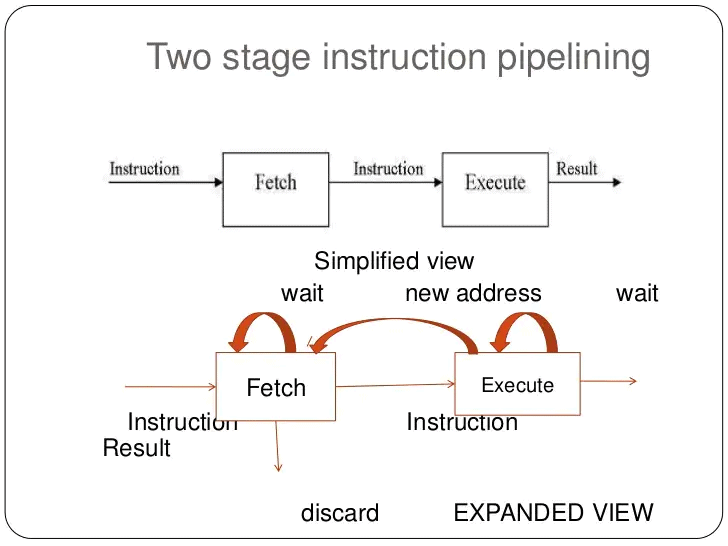• Above figure depicts this approach. The pipeline has two independent stages. The first stage fetches an instruction and buffers it. When the second stage is free, the first stage passes it the buffered instruction.
• While the second stage is executing the instruction, the first stage takes advantage of any unused memory cycles to fetch and buffer the next instruction. This is called instruction prefetch or fetch overlap.
• This process will speed up instruction execution only if the fetch and execute stages were of equal duration, the instruction cycle time would be halved.

However, if we look more closely at this pipeline, we will see that this doubling of execution rate is unlikely for 3 reasons:
(i) The execution time will generally be longer than the fetch time. Thus, the fetch stage may have to wait for some time before it can empty its buffer.
(ii) A conditional branch instruction makes the address of the next instruction to be fetched unknown. Thus, the fetch stage must wait until it receives the next instruction address from the execute stage. The execute stage may then have to wait while the next instruction is fetched.
(iii) When a conditional branch instruction is passed on from the fetch to the execute stage, the fetch stage fetches the next instruction in memory after the branch instruction. Then, if the branch is not taken, no time is lost. If the branch is taken, the fetched instruction must be discarded and a new instruction fetched.

To gain further speedup, the pipeline must have more stages. Let us consider the following decomposition of the instruction processing:

1. Fetch instruction (FI): Read the next expected instruction into a buffer.
2. Decode instruction (DI): Determine the opcode and the operand specifiers.
3. Calculate operands (CO): Calculate the effective address of each source operand. This may involve displacement, register indirect, indirect, or other forms of address calculation.
4. Fetch operands (FO): Fetch each operand from memory.
5. Execute instruction (EI): Perform the indicated operation and store the result, if any, in the specified destination operand location.
6. Write operand (WO): Store the result in memory.
• The below figure shows that a six-stage pipeline can reduce the execution time for 9 instructions from 54 time units to 14 time units.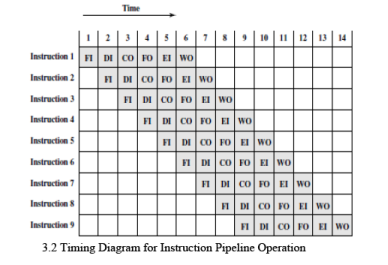• FO and WO stages involve memory access. If the six stages are not of equal duration, there will be some waiting involved at various pipeline stages. Another difficulty is the conditional branch instruction, which can invalidate several instruction fetches. A similar unpredictable event is an interrupt.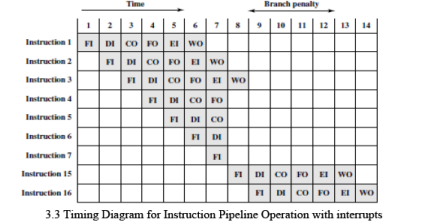• Figure 3.3 illustrates the effects of the conditional branch, using the same program as Figure 3.2. Assume that instruction 3 is a conditional branch to instruction 15. Until the instruction is executed, there is no way of knowing which instruction will come next. The pipeline, in this example, simply loads the next instruction in sequence (instruction 4) and proceeds.
• In Figure 3.2, the branch is not taken. In Figure 3.3, the branch is taken. This is not determined until the end of time unit 7.At this point, the pipeline must be cleared of instructions that are not useful. During time unit 8, instruction 15 enters the pipeline.
• No instructions complete during time units 9 through 12; this is the performance penalty incurred because we could not anticipate the branch. Figure 3.4 indicates the logic needed for pipelining to account for branches and interrupts.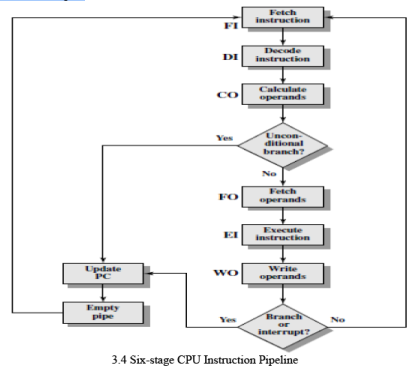• Figure 3.5 shows same sequence of events, with time progressing vertically down the figure, and each row showing the state of the pipeline at a given point in time.
• In Figure 3.5a (which corresponds to Figure 3.2), the pipeline is full at time 6, with 6 different instructions in various stages of execution, and remains full through time 9; we assume that instruction I9 is the last instruction to be executed.
• In Figure 3.5b, (which corresponds to Figure 3.3), the pipeline is full at times 6 and 7. At time 7, instruction 3 is in the execute stage and executes a branch to instruction 15. At this point, instructions I4 through I7 are flushed from the pipeline, so that at time 8, only two instructions are in the pipeline, I3 and I15.

For high-performance in pipelining designer must still consider about:

• At each stage of the pipeline, there is some overhead involved in moving data from buffer to buffer and in performing various preparation and delivery functions. This overhead can appreciably lengthen the total execution time of a single instruction.
• The amount of control logic required to handle memory and register dependencies and to optimize the use of the pipeline increases enormously with the number of stages. This can lead to a situation where the logic controlling the gating between stages is more complex than the stages being controlled.
• Latching delay: It takes time for pipeline buffers to operate and this adds to instruction cycle time.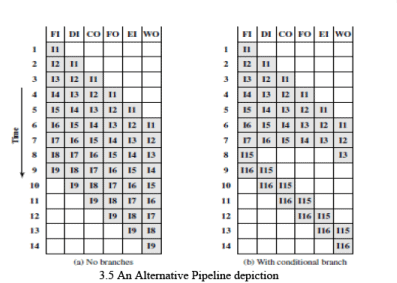Pipelining Performance

• Measures of pipeline performance and relative speedup: The cycle time t of an instruction pipeline is the time needed to advance a set of instructions one stage through the pipeline; each column in Figures 3.2 and 3.3 represents one cycle time.
• The cycle time can be determined as
t = max [ti] + d = tm + d 1 … i … k
where
ti = time delay of the circuitry in the ith stage of the pipeline
tm = maximum stage delay (delay through stage which experiences the largest delay)
k = number of stages in the instruction pipeline
d = time delay of a latch, needed to advance signals and data from one stage to the next
• In general, the time delay d is equivalent to a clock pulse and tm W d. Now suppose that n instructions are processed, with no branches. Let Tk,n be the total time required for a pipeline with k stages to execute n instructions. Then
Tk,n = [k + (n -1)]t
• A total of k cycles are required to complete the execution of the first instruction, and the remaining n -1 instructions require n -1 cycles.
• This equation is easily verified from Figures 3.1. The ninth instruction completes at time cycle 14:
14 = [6 + (9 -1)]
• Now consider a processor with equivalent functions but no pipeline, and assume that the instruction cycle time is kt. The speedup factor for the instruction pipeline compared to execution without the pipeline is defined as
sk = Tl,n/Tk,n = nkr/(k + (n -1)) * r = nk/(k + (n -1))
Offer running on EduRev: Apply code STAYHOME200 to get INR 200 off on our premium plan EduRev Infinity!

## Digital Electronics

92 videos|91 docs|12 tests

,

,

,

,

,

,

,

,

,

,

,

,

,

,

,

,

,

,

,

,

,

;